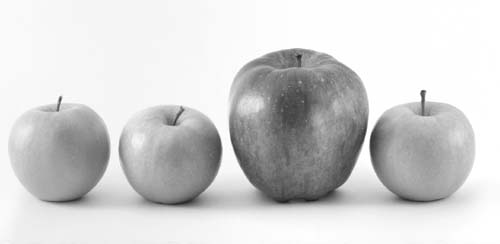NextPrevious

# What are proper and improper fractions?

The word “fraction” to most of us means a part of something; in mathematics, it represents a type of numeral, in most cases the quotient of two integers, with the top number called the numerator (the number of parts) and the bottom number the denominator (how many parts the whole is divided into). Written, the numerator and denominator are separated by a “/” or “—”. A fraction is usually denoted by a/b, in which “a” and “b” are whole numbers and “b” is not equal to zero (for the explanation of why you cannot divide by zero, see above).Proper fractions are used to express parts of a whole. For example, in this photo there are four apples but only one red apple. One in four, or 1/4, of the apples is a red apple. In an improper fraction, the numerator is larger than the denominator, such as 4/3 or 3/2.

A rational number between 0 and 1 can be represented by fractions (by the division of two numbers). If the quotient is less than one, such as ½ or 2/5, then it is called a proper fraction; if the quotient is greater than one—or in other words, if the numerator of a fraction is larger than the denominator, such as 23/7—it is called an improper fraction.

Close

This is a web preview of the "The Handy Math Answer Book" app. Many features only work on your mobile device. If you like what you see, we hope you will consider buying. Get the App